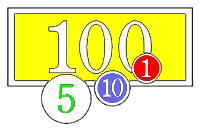# Rounding Numbers - Rules & Examples For Fractions & Sums

Updated February 21, 2017 | Factmonster StaffA rounded number has about the same value as the number you start with, but it is less exact.For example, 341 rounded to the nearest hundred is 300. That is because 341 is closer in value to 300 than to 400. When rounding off to the nearest dollar, \$1.89 becomes \$2.00, because \$1.89 is closer to \$2.00 than to \$1.00

### Rules for Rounding

Here's the general rule for rounding:

• If the number you are rounding is followed by 5, 6, 7, 8, or 9, round the number up. Example: 38 rounded to the nearest ten is 401
• If the number you are rounding is followed by 0, 1, 2, 3, or 4, round the number down. Example: 33 rounded to the nearest ten is 30

### What Are You Rounding to?

When rounding a number, you first need to ask: what are you rounding it to? Numbers can be rounded to the nearest ten, the nearest hundred, the nearest thousand, and so on.

Consider the number 4,827.

• 4,827 rounded to the nearest ten is 4,830
• 4,827 rounded to the nearest hundred is 4,800
• 4,827 rounded to the nearest thousand is 5,000

All the numbers to the right of the place you are rounding to become zeros. Here are some more examples:

• 34 rounded to the nearest ten is 30
• 6,809 rounded to the nearest hundred is 6,800
• 1,951 rounded to the nearest thousand is 2,000

### Rounding and Fractions

Rounding fractions works exactly the same way as rounding whole numbers. The only difference is that instead of rounding to tens, hundreds, thousands, and so on, you round to tenths, hundredths, thousandths, and so on.

• 7.8199 rounded to the nearest tenth is 7.8
• 1.0621 rounded to the nearest hundredth is 1.06
• 3.8792 rounded to the nearest thousandth is 3.879

Here's a tip: to avoid getting confused in rounding long decimals, look only at the number in the place you are rounding to and the number that follows it. For example, to round 5.3824791401 to the nearest hundredth, just look at the number in the hundredths place—8—and the number that follows it—2. Then you can easily round it to 5.38.

### Rounding and Sums

Rounding can make sums easy. For example, at a grocery store you might pick up items with the following prices:

• \$2.25
• \$0.88
• \$2.69

If you wanted to know about how much they would cost, you could add up the prices with a pen and paper, or try to add them in your head. Or you could do it the simple way—you could estimate by rounding off to the nearest dollar, like this:

• \$2.00
• \$1.00
• \$3.00

By rounding off, you could easily figure out that you would need about \$6.00 to pay for your groceries. This is pretty close to the exact number of \$5.82.

As you can see, in finding a round sum, it is quickest to round the numbers before adding them.

1. Some statisticians prefer to round 5 to the nearest even number. As a result, about half of the time 5 will be rounded up, and about half of the time it will be rounded down. In this way, 26.5 rounded to the nearest even number would be 26—it would be rounded down. And, 77.5 rounded to the nearest even number would be 78—it would be rounded up.

Sources +